Project tutorial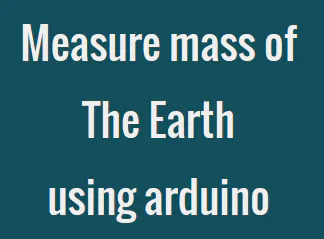# How to Measure Mass of the Earth Using Arduino © GPL3+

We live on a beautiful planet called earth, do you know how much is its weight? Lets find out.

• 6,226 views
• 20 respects

## Apps and online services

### Intro

One fine day, I was weighing my self on a weighing scale. Suddenly a thought came to my mind, 'How much would be the mass of Earth?' Leave that apart 'How can we even measure it?' There is no such weighing scale on which Earth can be placed. There has to be some indirect way to measure mass of the Earth. Here I present implementation of one such indirect method to measure mass of the Earth.

### Step 1: The Indirect Method

In early nineteenth century scientists used Newton's second law and Newton's law of universal gravitation to measure mass of the Earth. These equations are F = ma and F=(GmM)/(r^2) respectively(m=mass of the object, a=acceleration, G=Gravitational Constant, M=mass of the Earth, r=Radius of the earth). If we substitute g i.e. acceleration due to gravity for the 'a' acceleration term and combine these two equation we get.

mg = GmM/r^2

This equation can be solved for M i.e mass of the earth.

M = (gr^2)/G.

We will assume that we know the value of G and r. We will find g i.e. acceleration due to gravity using a small experiment, involving arduino and a few sensors. Finally we will put all things together to find the value of M.

look at the attached image for proper mathematical expressions.

Let's get started with the experiment.

### Step 2: Overview of the Experiment

(bear with me for a bit of math here arduino will kick in soon)

To determine acceleration due to gravity we need to know some thing called 'free fall', it is a motion in which a body/object moves downward towards earth, under the force of gravity, only. Now, there are few scientific terms that characterizes this free fall. They are initial velocity, final velocity, distance traveled, time of flight and acceleration.

• Initial velocity - velocity of the free falling body at the start of free fall or start of experiment.
• Final velocity - velocity of the free falling body at the end of free fall of end of experiment.
• Distance traveled - distance covered by the free falling body during free fall.
• Time of flight - time taken to cover distance traveled under free fall.
• Acceleration - the rate of change of velocity observed by body in free fall. It is equal to acceleration due to gravity.

Now, there is one of the Newton's equation that goes like -

Distance traveled = (initial velocity * time of flight) + (acceleration * (time of flight)^2)/2

There are 4 variables in above equation, including one of our interest i.e. 'acceleration', out of these 4 variables if we know 3 we can calculate the 4th remaining unknown variable.

We can fix 2 of them

• Distance traveled = 1 Meter (we will drop our object from 1 meter height)
• initial velocity = 0 m/s (we will start our experiment from rest)

We are left with 2 unknown variables, this is where arduino kicks in and helps us in finding time of flight. To accurately determine time of flight we need two time stamps 1. start of flight and 2. end of flight.

We will make custom circuitry to determine these two time stamps in step 4, I describe the circuitry to find time-stamp for start of flight and in step 5 end of flight.

With the help of arduino and few sensors we will calculate time of flight, leaving only one unknown in above equation i.e. acceleration due to gravity (bingoooo!!!).

Let us put our fixed variable values in the above mentioned equation, to further simplify the equation.

1 = (g * (time of flight)^2)/2

So,

g = 2 / (time of flight)^2

Details of the experiment will follow in step 7.

In case of any doubt refer the attached image.

### Step 3: Things we will Need

Here is the list of things we will need for experiment to measure acceleration due to gravity.

• Any Arduino board, preferably uno/duomilanove (no specific reason just that I have used this board for this experiment)
• USB cable for connecting Arduino to PC
• 560 Ohm resistors (4 in quantity), 100 Ohm resistors (2 in quantity)
• piezoelectric sensor (4 in quantity)
• One pair of IR Tx (IR LED) and Rx(Photodiode)
• One potentiometer
• One opamp (like UA741C)
• Three 1.2 meter long wires
• small 5cmx5cm general purpose PCB for assembling IR circuitry
• Tape
• Cardboard sheet

In case of any doubt look at the attached Images.

Step 4: Schematic 1: The IR Circuitry

This circuit will be used to time-stamp the starting time of free fall for an object used to measure 'g'. Here are the important points about the attached fritzing schematic image.

• IR LED is used in forward bias which acts as just normal LED in this application i.e. illuminating the IR receiver Photodiode.
• Photodiode is used in reverse bias.
• Output from the junction of cathode and resistor is connected to one of the input terminal of opamp.
• Other input terminal of opamp is connected to potentiometer that determines the threshold voltage.
• Output of opamp goes to the Arduino board.

Refer the video (time= 5m 16s) in case of ant doubt.

### Step 5: Schematic 2: The Piezoelectric Circuitry

This circuit will be used to time-stamp the ending time of free fall for an object used to measure 'g'. Here are the important points about the attached fritzing schematic image.

• 4 piezoelectric sensors are connected as shown in the schematic.
• 4 560 Ohm resistor are connected in parallel with the piezoelectric sensors to reduce the voltage spike generated by piezoelectric sensor when free falling object hits it's surface.
• Negative terminal of piezoelectric sensors is connected to circuit ground.
• Positive terminal of piezoelectric sensor is connected to analog input pins.

In case of doubt refer to attached video (time = 9m 2 sec).

### Step 6: Putting Things Together

Follow these instructions to put things together.

• Use card board to make roof like structure and stick it to the wall at around 1.1 meters.
• Attach IR module facing downward to cardboard roof.
• Connect output of opamp to pin no 4 of Arduino using one of the 1.2m long wires.
• Connect Vcc and GND from arduino to IR module using rest of the 1.2m long wires.
• Make sure that things are firmly attached wires to the wall, IR module to the cardboard roof, piezoelectric module to the ground just below IR module.

In case of doubt, look at the attached image, it is properly marked for ease of understanding the assembly.

### Step 7: Details of the Experiment

With all things at place (except Arduino code, discussed in step 8), lets go through the procedure of this experiment.

• Stick a small thin projection on top of the object used for the free fall as shown in the image numbered as 1.
• Before we leave our object to carry on free fall, hold it just at the 1 meter mark, such that projection on the top obstruct the light from IR to photo diode. As shown in the image numbered as 2.
• leave the object without imparting any force to let it fall free on the piezoelectric landing zone.
• As it falls down there is no obstruction in the path of IR TX and RX, giving us the signal about just left object in term of starting time of free fall.
• As object touches the landing zone, piezoelectric sensors generate controlled voltage spike notifying the arrival of object in term of ending time of the free fall.
• If we subtract ending time from the starting time we get time of flight that we will use to calculate the acceleration due to gravity as we discussed in step 2.

Step 8: Arduino Code

I am attaching the Arduino code for this experiment. Code is really simple. In case of any doubt refer the video where I explain the code in detail (time in video = 13minute 35 secs).

Step 9: Lets do the experiment

Following things should be taken care before doing this experiment.

• Threshold of the piezoelectric sensor should be properly set in the arduino code.
• Place piezoelectric panel just below the IR module.
• Make sure you drop the object from 1 Meter with out imparting any external force.

Here I am doing this experiment at time stamp 17 minute in the attached video. We will calculate Mass of earth using results i.e. time of flight obtained from this experiment.

Step 10: Results and mass of the Earth

After repeating this experiment for 3 times I got following results in terms of Time of Flight.

• 443 ms
• 443 ms
• 464 ms

Lets take average.

Average time of flight = 450 ms

If we substitute this value in equation to obtain acceleration due to gravity we get acceleration to be around 9.8755 m/s2. As seen in the attached image.

Finally substituting this value of 'g' to get mass of earth we get it to be around 6.0066 x 1024 Kg, as you can see in the attached image.

Actual mass of the Earth as per google is 5.972 x 1024 Kg. I think we got close.

Thank you for your time, In case of any doubt refer the video or put it in the comments I will be happy to help.

## Code

##### Arduino codeArduino
simple arduino code
```const int sensorPin1 = A0;
const int sensorPin2 = A1;
const int sensorPin3 = A2;
const int sensorPin4 = A3;
const int photoPin = 4;
const int ledPin = 13;
const int threshold = 110;
char stringToPrint;

int startTime,endTime;

int val1,val2,val3,val4;

void setup() {
Serial.begin(115200);
// put your setup code here, to run once:
pinMode(ledPin,OUTPUT);
pinMode(sensorPin1,INPUT);
pinMode(sensorPin2,INPUT);
pinMode(sensorPin3,INPUT);
pinMode(sensorPin4,INPUT);
pinMode(photoPin,INPUT);
}

void loop() {
// put your main code here, to run repeatedly:

startTime = millis();
if(start == HIGH)
digitalWrite(ledPin,HIGH);
while(start == LOW)
{
digitalWrite(ledPin,LOW);
//sprintf(stringToPrint,"%d,%d,%d,%d",val1,val2,val3,val4);
//Serial.println(stringToPrint);
if((val1 >= threshold) || (val2 >= threshold) || (val3 >= threshold) || (val4 >= threshold))
{
endTime = millis();
sprintf(stringToPrint,"%d ms",endTime - startTime);
Serial.println(stringToPrint);
digitalWrite(ledPin,HIGH);
delay(1000);
digitalWrite(ledPin,LOW);
start = HIGH;
}
}
}
```

## Schematics

fritzing schematic for IR circuitary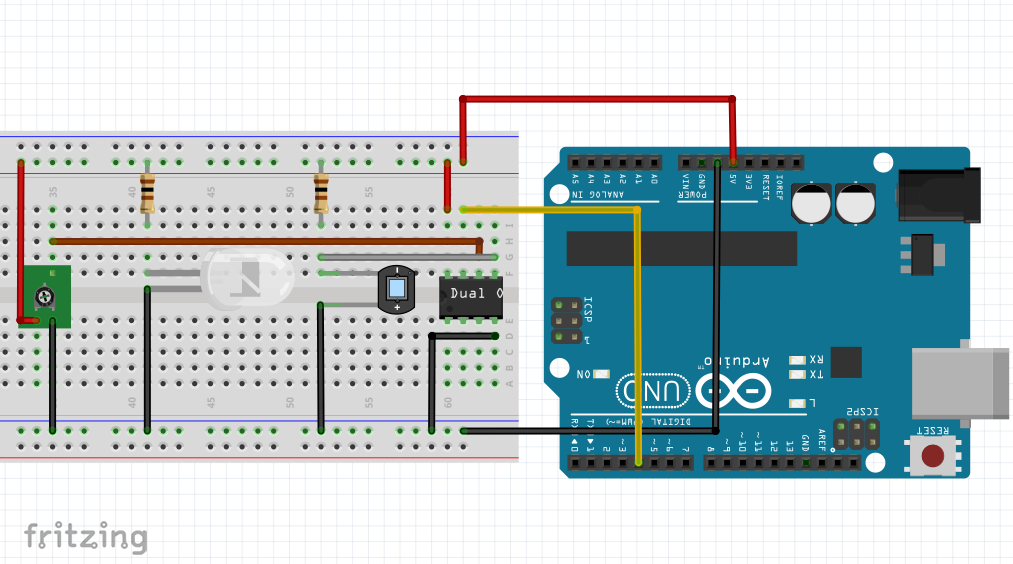hardware image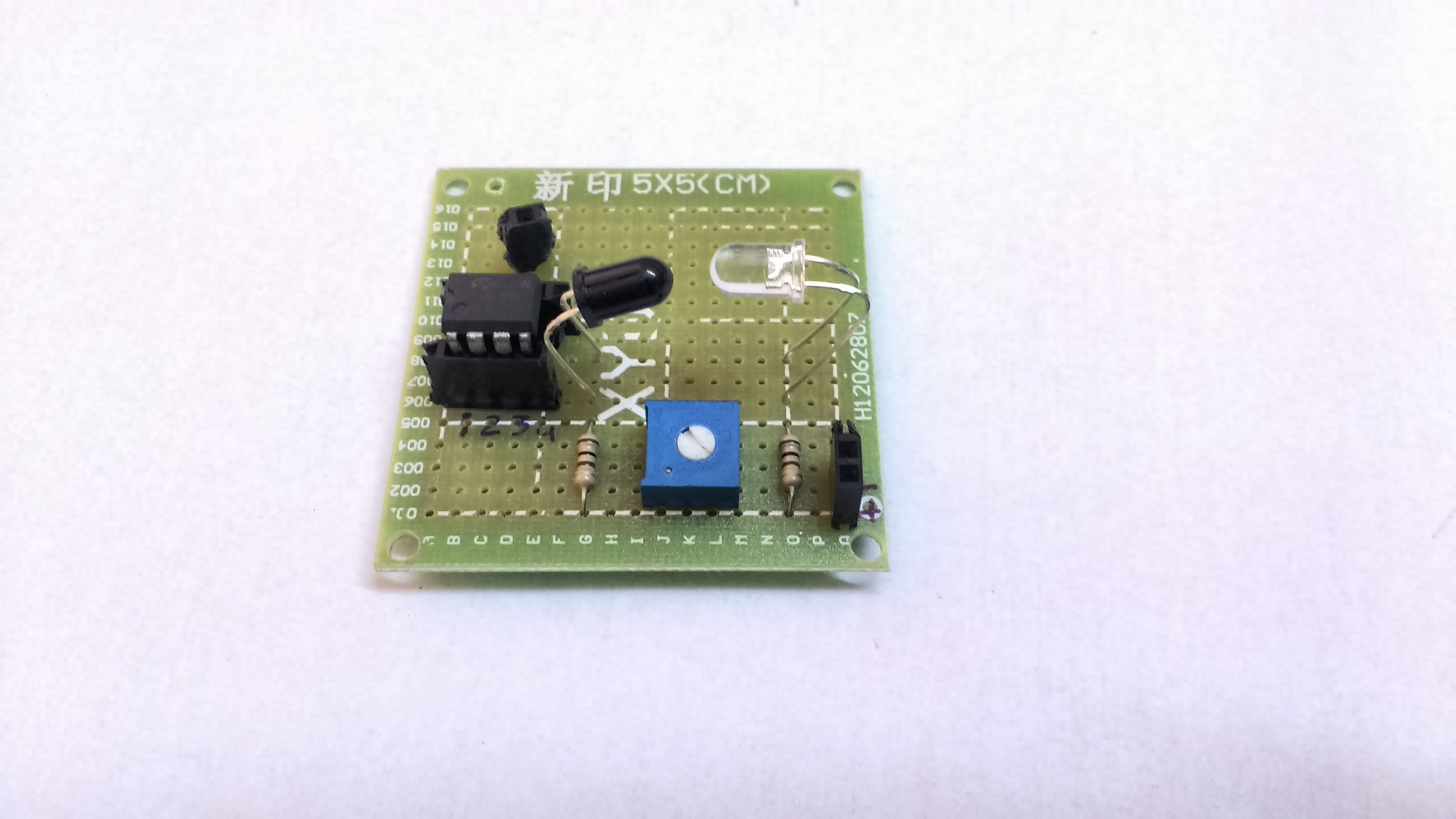fritzing schematic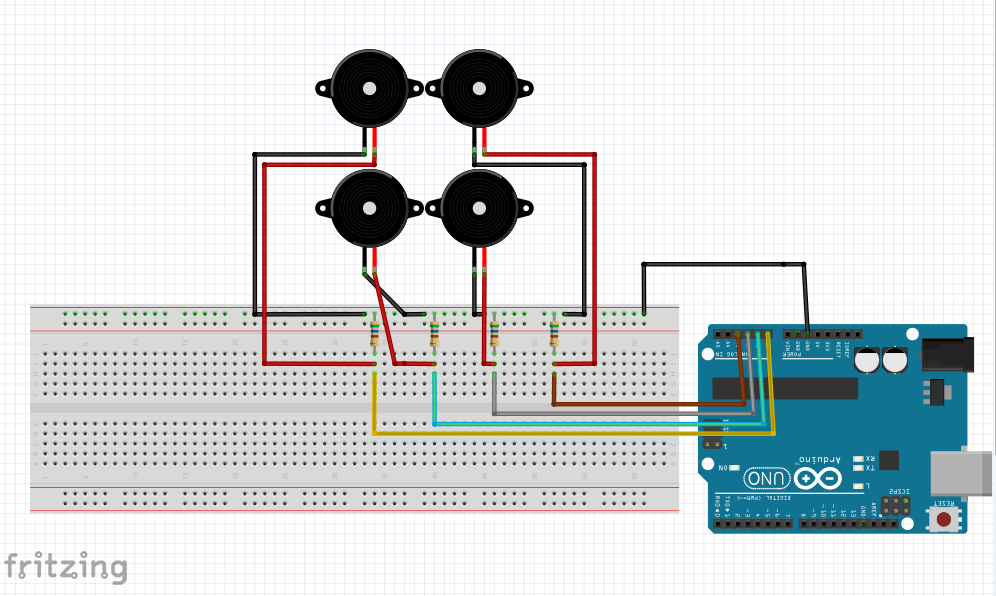hardware image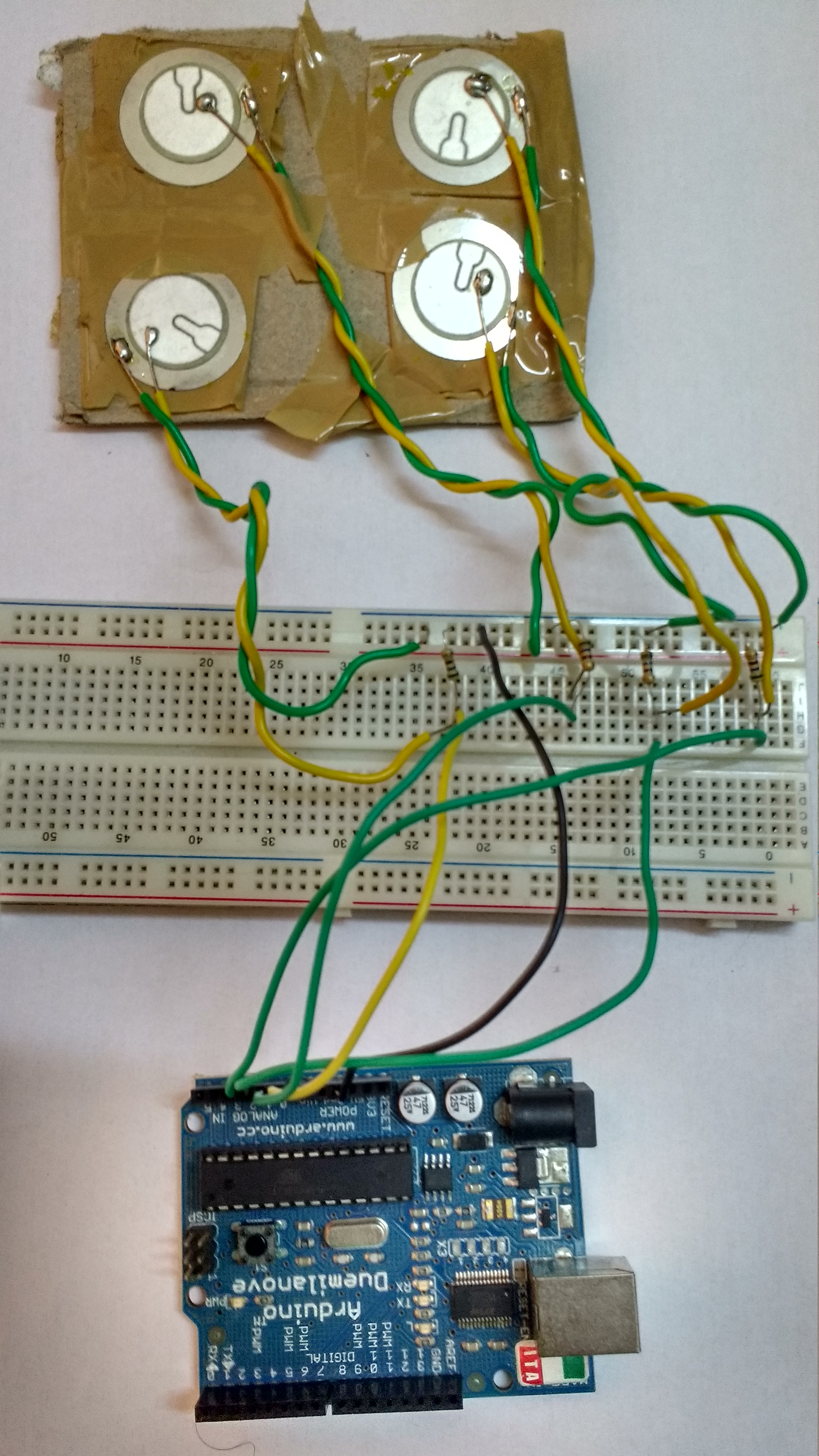#### Similar projects you might like

Project tutorial by Jayraj Desai

• 33,178 views
• 35 respects

#### GameBox - The Arduino LCD Console

Project tutorial by Team Mattia Colasanto

• 4,280 views
• 9 respects

#### Simple Programmable Robotic Arm

Project showcase by Ryan Chan

• 99,647 views
• 256 respects

#### Room temperature sensor with display on Mobile

Project showcase by Kaustubh Agarwal

• 30,170 views
• 63 respects